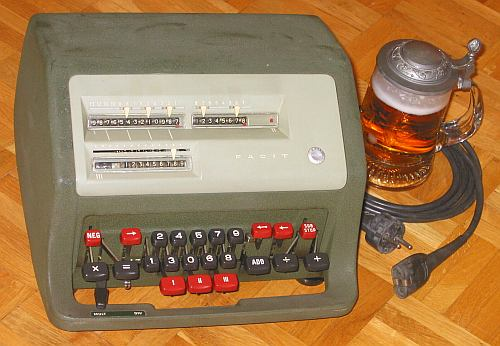previous <<==>> next

FACIT   CA1-13Observe the unusual (female) plug with diagonal slots (*)

Have a look inside
 ``` TECHNICAL DATA of the Electro-Mechanical Calculator FACIT CA1-13 **************************************************************************** Serial Number: 636312 (= Outside; Inside: 223539 = back ; 37(8) = top) Dimensions: (ca.) Width = 11_1/2 " / 29 cm Depth = 11 " / 28 cm Height = 7_1/2 " / 19 cm Weight: (ca.) 28_1/2 lbs / 13 kg Power Supply: 220 V / 70 W AC Mechanics: 10-Keyboard (DALTON type) Serial-to-parallel conversion with Principle: Pin-Wheel / Sprossenrad Special: FACIT's Solution Functions: Add, Subtract, Multiply, Divide Full-motorized Arithmetic! Registers: I Arithmetic = 13 Decimals II Counter = 8 Decimals III Input = 9 Decimals Hidden Memory = 8 Decimals Manufacturer: FACIT ( with a » FACIT DUESSELDORF GERMANY « seal ) Atvidaberg Sweden 1957 HOW TO USE the FACIT CA1-13 ***************************** B A S I C S : ============= o CLEARING REGISTERS with the red keys [I], [II] & [III] o ,,..,,,.. KEYS for serial input (left-to-right) o The actual POSITION of the INPUT UNIT is marked with a red arrow-pair refering to registers I & II (pointing the least significant digit) o [ADD] ADDS the entered number to the content of the arithmetic unit and CLEARS the input o [ + ] CONTINUOUS ADDITION as long as the key is pressed; when released: STEP-TO-LEFT, IF MAIN SWITCH is set: o MAIN SWITCH (down left) controls * COUNTING DIRECTION and * STEPPING: - Left »MULT«-Position: Stepping right-to-left - Right »DIV«-Position: Stepping left-to-right - Middle Position: IF the (right beside) SILVER LEVER is LEFT, THAN stepping NO; IF the SILVER LEVER is (pushed & shift) RIGHT, THAN stepping left-to-right. o [NEG] COUNTING DIRECTION (Toggle key) = NEGATIVE when (RED) mark visible (right of the counter) = POSITIVE when (BLK) mark visible o [ <-- ] STEP INPUT UNIT to the LEFT o [ <-o ] JUMP INPUT UNIT to the LEFT (with ZEROS on END) o [ --> ] STEP INPUT UNIT to the RIGHT o [-:-] CONTINUOUS SUBTRACTION as long as the key is pressed; when released: STEP-TO-RIGHT, IF MAIN SWITCH is set to MIDDLE position AND the SILVER LEVER is RIGHT o [ x ] for MULTIPLICATION: After entered [x] STORES the 1st number in the hidden MEMORY o [ = ] for MULTIPLICATION: After entered the 2nd number [=] STARTS the multiplication o [SUB-STOP] has two functions: * PRESSED with [-:-] key: CLEARS input register after subtraction * INTERRUPTS a looping division; ALTERNATIVE: Press-and-hold [-:-] STOPS DIVISION when enough digits are calculated ADDITION & SUBTRACTION: ======================= Example: 123 + 45 - 6 = 162 Clear: [I], [II], [III]; Set: MAIN SWITCH to MIDDLE position; Counting Direction (BLK) positive ADD: Enter the first number (123) into input register (III). Press the [ADD] key to transfer the input into arithmetic unit (I). The counting unit (II) displays the figure 1. Enter the second number (45). Press the [ADD] key to add the number. The arithmetic unit displays the inter- mediate sum (168) and the counting unit displays the figure 2. SUBTRACT: Enter the third number (6) into input unit and press the [-:-] key ( !!! TOGETHER !!! ) with the [SUB-STOP] key. The arithmetic unit displays the result (162) and the counting unit is decreased by 1. REMARK: NEGATIVE RESULTS are displayed in the arithmetic unit as the COMPLEMENT of the next higher 10, 100, 1000, ... Example: -12 = 99...9988 MULTIPLICATION: =============== Example: 123 x 45 = 5535 Clear: [I], [II], [III]; Set: MAIN SWITCH to LEFT »MULT«-Position; Counting Direction (BLK) positive Enter the multiplicand (123) into input unit. Press the [x] key and the number goes in the hidden memory. Enter the multiplicator (45). Press [=] and the multiplication will start automatically. When done: The multiplicand (123) stays in the counter unit, the multiplicator (45) is in the input unit and the result (5535) is in the arithmetic unit. MULTIPLICATION with a CONSTANT: =============================== Example: 123 x 45 = 5535 123 x 67 = 8241 123 x 89 = 10947 Clear: [I], [II], [III]; Set: MAIN SWITCH to LEFT »MULT«-Position; Counting Direction (BLK) positive Enter the 1st factor (123) and press [x]. Enter the 2nd factor (45) and press [=]. The result (5535) is in the arithmetic unit. Press [x], [I], [II]; Enter the new factor (67) and press [=]. The result (8241) is in the arithmetic unit. Press [x], [I], [II]; Enter the new factor (89) and press [=]. The result (10947) is in the arithmetic unit. The CONSTANT Factor (123) stays in the counter unit, factor (89) in the input unit. SQUARE: ======= Example: 123 x 123 = 15129 Clear: [I], [II], [III]; Set: MAIN SWITCH to LEFT »MULT«-Position; Counting Direction (BLK) positive Enter the number (123), press [=] and read the result (15129). The 1st factor (123) stays in counter, 2nd factor (123) in input unit. DIVISION: ========= Example: 22 : 7 = 3.1428571 Remainder 3 Clear: [I], [II], [III]; Set: MAIN SWITCH to RIGHT »DIV«-Position; Counting Direction (RED) negative Enter the dividend (22) into input unit. Press JUMP [<-o] key; Press [ADD]. Enter the divisor (07) into input unit. Press JUMP [<-o] key; Press [-:-] and the division will start automatically. The result (3.1428571) is in the counter unit, and the remainder (3..) is in the arithmetic unit. The divisor (7..) stays in the input unit, therefore an additional decimal can be estimated ... ( 4, cause 4 x 7 = 28). Have a look at "Calculating Trickies" ... (*) !! see FAQs: The old GERMAN POWER PLUG for 220 VAC FITS !! impressum: **************************************************************************** © C.HAMANN http://public.BHT-Berlin.de/hamann 09/12/07 ```